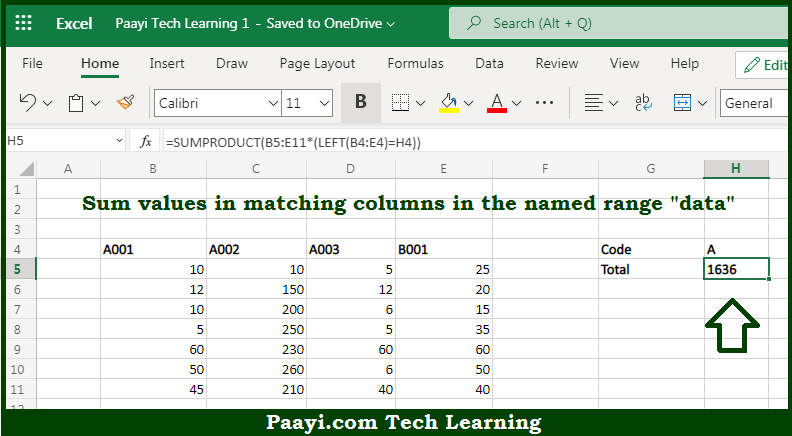# Learn How to SUM Matching Columns in Microsoft Excel

Written by | 0 Comments | 533 Views

In this article, you will learn how to SUM various things in Microsoft Excel using a single/combination(s) of functions. You will also know how to SUM Matching Columns and see the generic formula.

SUM Matching Columns in Microsoft Excel

The main purpose of this formula is to sum values in columns by matching column headers. Here we will learn how to sum matching columns in the workbook in Microsoft Excel. That implies, with the help of a formula based on the SUMPRODUCT function you can able sum values in columns by matching column headers. So, with the help of this formula, you can able to sum matching columns in the workbook in Microsoft Excel.

General Formula to SUM Matching Columns

The Explanation for the SUM Matching ColumnsSo we know that with the help of the given formula above you can able to sum values in columns by matching column headers. Here we will learn how to sum matching columns in the workbook in Microsoft Excel. As we know that the formula provided here is based on the SUMPRODUCT function to sum values in matching columns in the named range "data". If all data were provided to the SUMPRODUCT function in a single range, the result would be the sum of all values in the range. So, with the help of this formula, you can able to sum matching columns in the workbook in Microsoft Excel.